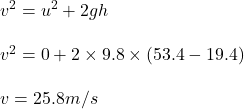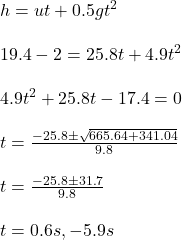## A cement block accidentally falls from rest from the ledge of a 53.4-m-high building. When the block is 19.4 m above the ground, a man, 2.00

Question

A cement block accidentally falls from rest from the ledge of a 53.4-m-high building. When the block is 19.4 m above the ground, a man, 2.00 m tall, looks up and notices that the block is directly above him. How much time, at most, does the man have to get out of the way

in progress 0
6 months 2021-07-17T23:27:24+00:00 1 Answers 1 views 0

The time required by the man to get out of the way is 0.6 s.

Explanation:

height of building, H = 53.4 m

height of block, h = 19.4 m

height of man, h’ = 2 m

Let the velocity of the block at 19.4 m is v.

use third equation of motionNow let the time is t.

Use second equation of motionTime cannot be negative so time t = 0.6 s.